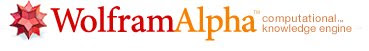## Monday, May 18, 2009

### Wolfram Alpha: a revolutionary answer-engineWolfram Alpha, an answer-engine developed by the British mathematician and physicist Stephen Wolfram and his team from Wolfram Research, was launched last Friday. A lot of people are considering Wolfram Alpha a Google-killer, but it isn't. They have different proposals. Google is a search engine and Wolfram Alpha is a computational knowledge engine. Wolfram Alpha uses built-in models of fields of knowledge, with algorithms and growing amount of data to calculate its responses. Wolfram Alpha is built on Wolfram's earlier flagship product, Mathematica.

According the site of Wolfram Alpha:
"Making the world's knowledge computable - Today's WolframAlpha is the first step in an ambitious, long-term project to make all systematic knowledge immediately computable by anyone. You enter your question or calculation, and WolframAlpha uses its built-in algorithms and growing collection of data to compute the answer."The Wolfram Alpha's Goal:
"WolframAlpha's long-term goal is to make all systematic knowledge immediately computable and accessible to everyone. We aim to collect and curate all objective data; implement every known model, method, and algorithm; and make it possible to compute whatever can be computed about anything. Our goal is to build on the achievements of science and other systematizations of knowledge to provide a single source that can be relied on by everyone for definitive answers to factual queries."

The Wolfram Alpha' Status:
"As of now, WolframAlpha contains 10+ trillion of pieces of data, 50,000+ types of algorithms and models, and linguistic capabilities for 1000+ domains. Built with Mathematica—which is itself the result of more than 20 years of development at Wolfram Research—WolframAlpha's core code base now exceeds 5 million lines of symbolic Mathematica code. Running on supercomputer-class compute clusters, WolframAlpha makes extensive use of the latest generation of web and parallel computing technologies, including webMathematica and gridMathematica."

Wolfram Alpha is really impressive and I think it can revolutionize the way how we use the web to find information.

Video tour of the Wolfram Alpha control room at the launch, recorded by Nova Spivack:

Nova Spivack also wrote a great article about Wolfram Alpha entitled Wolfram Alpha is Coming -- and It Could be as Important as Google (But It's Completely Different)